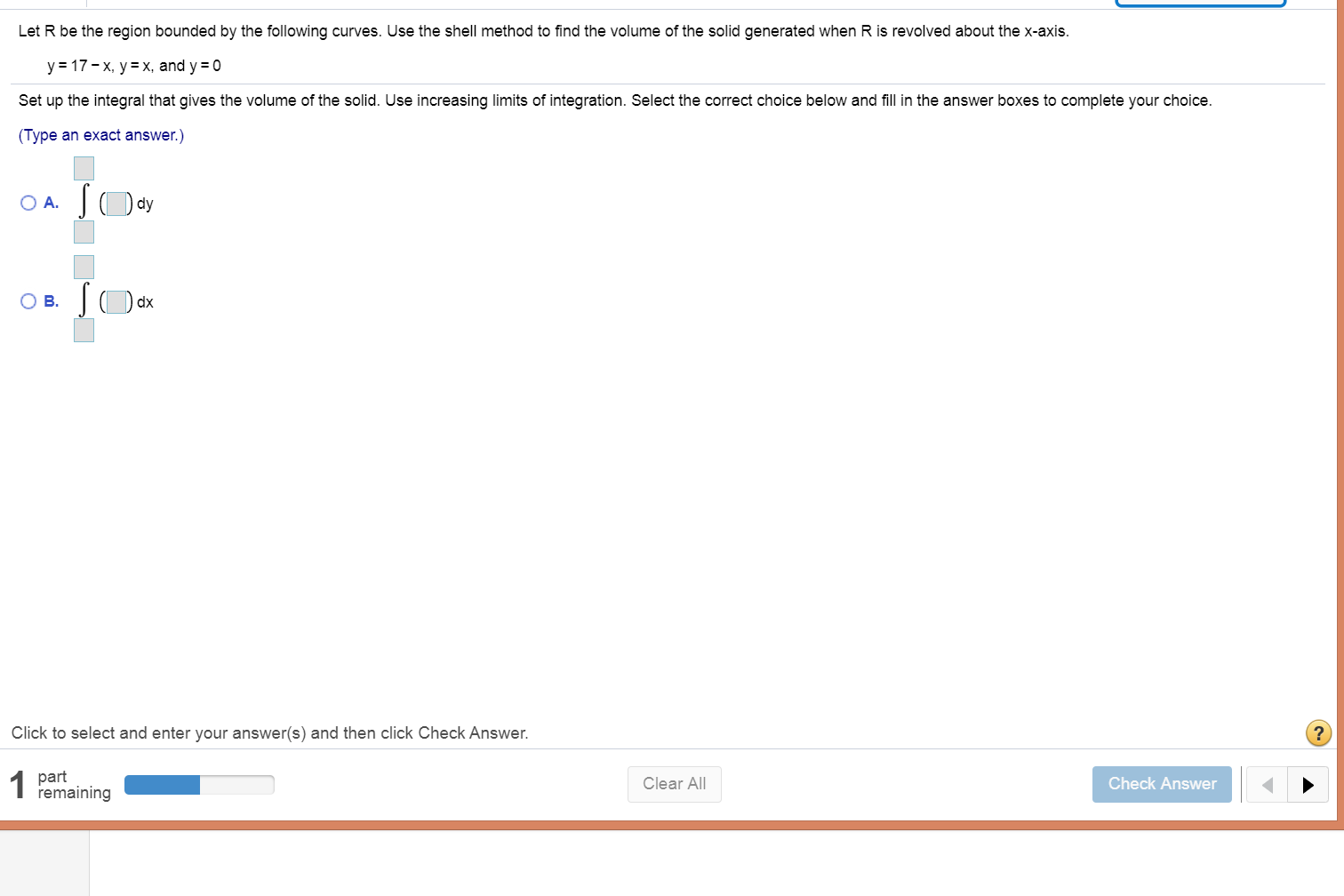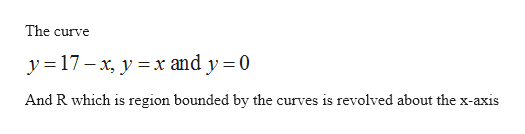# Let R be the region bounded by the following curves. Use the shell method to find the volume of the solid generated when R is revolved about the x-axisy = 17-x, yx, and y = 0Set up the integral that gives the volume of the solid. Use increasing limits of integration. Select the correct choice below and fill in the answer boxes to complete your choice.(Type an exact answer.)O A.Ов.dxClick to select and enter your answer(s) and then click Check Answer.?1partremainingClear AllCheck Answer

Question
10 viewshelp_outlineImage TranscriptioncloseLet R be the region bounded by the following curves. Use the shell method to find the volume of the solid generated when R is revolved about the x-axis y = 17-x, yx, and y = 0 Set up the integral that gives the volume of the solid. Use increasing limits of integration. Select the correct choice below and fill in the answer boxes to complete your choice. (Type an exact answer.) O A. Ов. dx Click to select and enter your answer(s) and then click Check Answer. ? 1part remaining Clear All Check Answer fullscreen
check_circle

Step 1

Given thathelp_outlineImage TranscriptioncloseThe curve y 17-x, y x and y 0 And R which is region bounded by the curves is revolved about the x-axis fullscreen
Step 2

To find volume of the solid generated by the region R using shall method

Step 3

### Want to see the full answer?

See Solution

#### Want to see this answer and more?

Solutions are written by subject experts who are available 24/7. Questions are typically answered within 1 hour.*

See Solution
*Response times may vary by subject and question.
Tagged in

### Calculus